# High School Chemistry : Using Acid Dissociation Constant (Ka)

## Example Questions

### Example Question #1 : Using Acid Dissociation Constant (Ka)

What is the concentration of hydronium ions in a solution if the hydroxide ion concentration is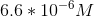?

Possible Answers: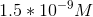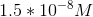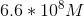Correct answer:Explanation:

For every acidic or basic solution, the product of the hydroxide ion concentration and the hydronium ion concentration will be equal to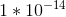, the dissociation constant for water. In other words: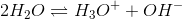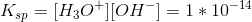Since we are given the hydroxide ion concentration, we can determine the hydronium ion concentration using this equation.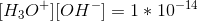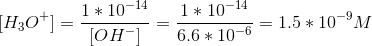### Example Question #2 : Using Acid Dissociation Constant (Ka)

A student has a 0.50M solution of acetic acid. She adds solid sodium acetate until the concentration of sodium acetate is 0.050M. What is the final pH of the solution?

The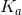for acetic acid is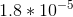. Assume the volume remains constant.

Possible Answers: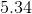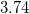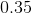Correct answer:Explanation:

Acetic acid is a weak acid, and its dissociation reaction is written as: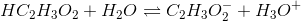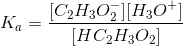Using an ICE table, we can find the pH of the solution when the sodium acetate is added:

I: There is initially a 0.50M concentration of acetic acid. Because sodium acetate will dissolve completely, there will be a 0.050M concentration of acetate ions in the solution.

C: As acetic acid dissociates, the concentrations of acetate ions and hydronium ions will increase by an unknown concentration. Conversely, the acetic acid concentration will decrease by the same amount.

E: By setting the equilibrium expression equal to the acid equilibrium constant, we can solve for the value of.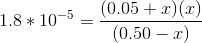*Because the value forwill be so much lower than the initial concentration, we can omit it from the acetate expression as well as the acetic acid concentration: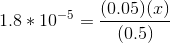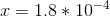Keep in mind thatis equal to the concentration of hydronium ions now in the solution.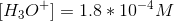Use this value in the equation for pH: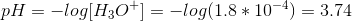### Example Question #3 : Using Acid Dissociation Constant (Ka)

What property distinguishes a strong acid from a weak acid?

Possible Answers:

There is no difference between a strong acid and a weak acid based on their properties in water

A weak acid will fail to dissociate completely in water and have a high Ka value

A strong acid will dissociate completely in water and have a low Ka value

A weak acid will dissociate completely in water and have a low Ka value

A strong acid will dissociate completely in water and have a high Ka value

Correct answer:

A strong acid will dissociate completely in water and have a high Ka value

Explanation:

The acid dissociation constant (Ka) is used to distinguish strong acids from weak acids. Strong acids have exceptionally high Ka values.

The Ka value is found by looking at the equilibrium constant for the dissociation of the acid. The higher the Ka, the more the acid dissociates. Thus, strong acids must dissociate more in water. In contrast, a weak acid is less likely to ionize and release a hydrogen ion, thus resulting in a less acidic solution.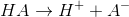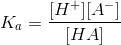This equation makes it clear that the more the acid converts from its original form to its ionzied, dissociated form, the higher the Ka value will be.

### Example Question #4 : Using Acid Dissociation Constant (Ka)

Which of the following is what determines the strength of an acid?

Possible Answers:

The Kb

The Ka

How many bonds the central atom makes

Its physical state

Electronegativity values

Correct answer:

The Ka

Explanation:

The Ka is the acid dissociation constant, and thus it is what determines how strong the acid is. Stronger acids dissociate to a greater extent and produce lower pH values.

### Example Question #1 : Using Acid Dissociation Constant (Ka)

Determine the equilibrium concentration of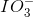ions in a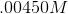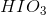solution.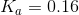Possible Answers: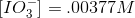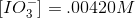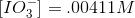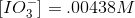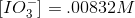Correct answer:Explanation:

The definition offor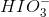is: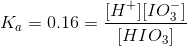Set up an "ICE" table: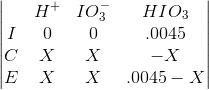Plug in values: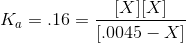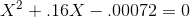Use the quadratic formula to solve: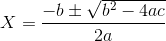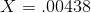### Example Question #1 : Using Acid Dissociation Constant (Ka)

Determine the equilibrium concentration of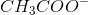ions in a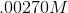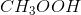solution.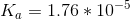Possible Answers: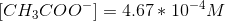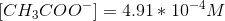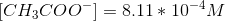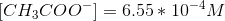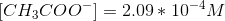Correct answer:Explanation: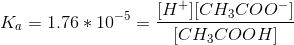Set up an "ICE" table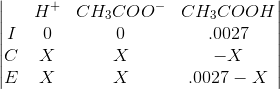Plug in values: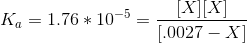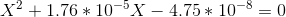Use the quadratic formula: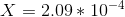### All High School Chemistry Resources